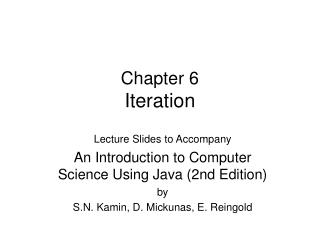DownloadDownload PresentationChapter 6 Iteration

Chapter 6 Iteration

Download PresentationChapter 6 Iteration

- - - - - - - - - - - - - - - - - - - - - - - - - - - E N D - - - - - - - - - - - - - - - - - - - - - - - - - - -
Presentation Transcript

1. Chapter 6Iteration Lecture Slides to Accompany An Introduction to Computer Science Using Java (2nd Edition) by S.N. Kamin, D. Mickunas, E. Reingold

2. Chapter Preview In this chapter we will: • discuss the use of repetition (iteration) in programming algorithms • describe the Java while, for, and do-while statements • demonstrate the use of loop invariants to verify the correctness of loops • show the use of loops in data entry and computer animation

3. while Loop • Repeated executes body of the loop which can be a single or compound statement • A while loop will execute as long as its condition is true • An infinite loop will occur if the condition never becomes false • Example: count = 1; while (count <= 10) count = count + 1;

4. while Loop From Temperature Conversion Program OutputBox out = new OutputBox( ); out.println(“\tDEGREES C\tDEGREES F”); // initialize the loop variable cent = LOW_TEMP; // test the loop variable while (cent <= HIGH_TEMP) { // convert C to F fahr = (9.0 / 5.0) * cent + 32.0; out.println(“\t” + cent “\t\t” fahr); // increment loop variable cent = cent + 1.0; }

5. for Statement • Used to implement pre-test loops that are controlled by incrementing a variable each time the loop is executed • Loop variable computations for initialization, testing, and incrementing appear in a single statement • Example: for(count=1; count <= 10; count = count + 1);

6. Done using for for ( statement1; condition; statement2 ) statement 3; Equivalent while loop statement1; while (condition) { statement3; statement2; } Comparing for and while

7. Temperature Conversion ProgramLoop Implemented Using for OutputBox out = new OutputBox( ); out.println(“\tDEGREES C\tDEGREES F”); for (cent = LOW_TEMP; cent <= HIGH_TEMP; cent = cent + 1.0;) { // convert C to F fahr = (9.0 / 5.0) * cent + 32.0; out.println(“\t” + cent “\t\t” fahr); }

8. do-while Statement • Used to implement post-test loops that always execute the loop body at least one time • A do-while loop will continue execute as long as its condition is true • An infinite loop will occur if the condition never becomes false • Example: count = 1; do { count = count + 1; } while (count <= 10);

9. Done using do-while do statement; while (condition); Equivalent while loop statement; while (condition) statement; Comparing do-while and while

10. Done using do-while numberOfDigits = 0; rest = number; do { rest = rest / 10; numberOfDigits++; } while (rest != 0); Equivalent while loop numberOfDigits = 0; rest = number; if (number = 0) numberOfDigits = 1; else while (rest > 0) { rest = rest / 10; numberOfDigits++; }; Comparing do-while and while

11. Done using do-while read score if ( not end of input) do { process score read score } while ( not end of input ); Done using while read score while ( not end of input ) { process score read score }; Reading Input Using a Loop

12. Sentinel Controlled Loop int i = 0; InputBox in = new InputBox(); In.setPrompt(“Enter data or –1 to terminate”); // read first score score = in.readInt(); while ( score != -1 ) { i++; // insert code to process score // read next score score = in.readInt(); }

13. Reading to End of Input int i = 0; InputBox in = new InputBox(); In.setPrompt(“Enter score (empty input ends data”); // read first score score = in.readInt(); while ( !in.eoi() ) { i++; // insert code to process score // read next score score = in.readInt(); }

14. Done using while read score while ( !in.eoi( ) ) { process score read score }; Done using do-while do { read score if ( !in.eoi( ) ) process score } while ( !in.eoi( ) ); Loop-and-a-Half

15. Done using do-while do { read if ( in.eoi( ) ) return process } while ( true ); Done using while while (true){ read if ( in.eoi( ) ) return process score }; Infinite Loop with Escape

16. Drawing in Java // code to read and display GIF image from file import java.awt.*; Import CSLib.*; … DrawingBox g = new DrawingBox(); … Toolkit tools = Toolkit.getDefaultToolkit(): Image mouse = tools.getImage(“C:/KMR/images/mouse.gif”); g.drawImage(mouse, x, y);

17. Nested Loops int advance = 0; // Draw clock and read input repeatedly do { for (int i = 1; i <= advance; i++) { // Advance time c.setMinute(c.getMinute()+1); // Update clock display d.clear(); c.display(d, SIZE/2, SIZE/2, SIZE/2); } in.setPrompt(“Advance how many minutes?”); advance = in.readInt(); } while (!in.eoi());